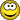Only RAM comparison failure between NestedCall.out and NestedCall.cmpClassicListThreaded3 messagesOpen this post in threaded view
|

NestedCall.out

| RAM | RAM | RAM | RAM | RAM | RAM | RAM |
|    261 |    261 |    256 |   4000 |   5000 |    135 |   5041 |

NestedCall.cmp

| RAM | RAM | RAM | RAM | RAM | RAM | RAM |
|    261 |    261 |    256 |   4000 |   5000 |    135 |    246 |

My NestedCall.asm is:

@256
D=A
@SP
M=D

@ret.0
D=A
@SP
A=M
M=D
@SP
M=M+1
@LCL
D=M
@SP
A=M
M=D
@SP
M=M+1
@ARG
D=M
@SP
A=M
M=D
@SP
M=M+1
@THIS
D=M
@SP
A=M
M=D
@SP
M=M+1
@THAT
D=M
@SP
A=M
M=D
@SP
M=M+1
@5
D=A
@SP
D=M-D
@0
D=D-A
@ARG
M=D
@SP
D=M
@LCL
M=D
@Sys.init
0;JMP
(ret.0)

(Sys.init)
@4000
D=A
@SP
A=M
M=D
@SP
M=M+1

@SP
M=M-1
A=M
D=M
@THIS
M=D

@5000
D=A
@SP
A=M
M=D
@SP
M=M+1

@SP
M=M-1
A=M
D=M
@THAT
M=D

@Sys.init\$ret.0
D=A
@SP
A=M
M=D
@SP
M=M+1
@LCL
D=M
@SP
A=M
M=D
@SP
M=M+1
@ARG
D=M
@SP
A=M
M=D
@SP
M=M+1
@THIS
D=M
@SP
A=M
M=D
@SP
M=M+1
@THAT
D=M
@SP
A=M
M=D
@SP
M=M+1
@5
D=A
@SP
D=M-D
@0
D=D-A
@ARG
M=D
@SP
D=M
@LCL
M=D
@Sys.main
0;JMP
(Sys.init\$ret.0)

@SP
M=M-1
A=M
D=M
@6
M=D

(Sys.init\$LOOP)

@Sys.init\$LOOP
0;JMP

(Sys.main)
@0
D=A
@SP
A=M
M=D
@SP
M=M+1

@0
D=A
@LCL
D=D+M
@R13
M=D
@SP
M=M-1
A=M
D=M
@R13
A=M
M=D

@0
D=A
@SP
A=M
M=D
@SP
M=M+1

@1
D=A
@LCL
D=D+M
@R13
M=D
@SP
M=M-1
A=M
D=M
@R13
A=M
M=D

@0
D=A
@SP
A=M
M=D
@SP
M=M+1

@2
D=A
@LCL
D=D+M
@R13
M=D
@SP
M=M-1
A=M
D=M
@R13
A=M
M=D

@0
D=A
@SP
A=M
M=D
@SP
M=M+1

@3
D=A
@LCL
D=D+M
@R13
M=D
@SP
M=M-1
A=M
D=M
@R13
A=M
M=D

@0
D=A
@SP
A=M
M=D
@SP
M=M+1

@4
D=A
@LCL
D=D+M
@R13
M=D
@SP
M=M-1
A=M
D=M
@R13
A=M
M=D

@4001
D=A
@SP
A=M
M=D
@SP
M=M+1

@SP
M=M-1
A=M
D=M
@THIS
M=D

@5001
D=A
@SP
A=M
M=D
@SP
M=M+1

@SP
M=M-1
A=M
D=M
@THAT
M=D

@200
D=A
@SP
A=M
M=D
@SP
M=M+1

@1
D=A
@LCL
D=D+M
@R13
M=D
@SP
M=M-1
A=M
D=M
@R13
A=M
M=D

@40
D=A
@SP
A=M
M=D
@SP
M=M+1

@2
D=A
@LCL
D=D+M
@R13
M=D
@SP
M=M-1
A=M
D=M
@R13
A=M
M=D

@6
D=A
@SP
A=M
M=D
@SP
M=M+1

@3
D=A
@LCL
D=D+M
@R13
M=D
@SP
M=M-1
A=M
D=M
@R13
A=M
M=D

@123
D=A
@SP
A=M
M=D
@SP
M=M+1

@Sys.main\$ret.1
D=A
@SP
A=M
M=D
@SP
M=M+1
@LCL
D=M
@SP
A=M
M=D
@SP
M=M+1
@ARG
D=M
@SP
A=M
M=D
@SP
M=M+1
@THIS
D=M
@SP
A=M
M=D
@SP
M=M+1
@THAT
D=M
@SP
A=M
M=D
@SP
M=M+1
@5
D=A
@SP
D=M-D
@1
D=D-A
@ARG
M=D
@SP
D=M
@LCL
M=D
0;JMP
(Sys.main\$ret.1)

@SP
M=M-1
A=M
D=M
@5
M=D

@0
D=A
@LCL
A=D+M
D=M
@SP
A=M
M=D
@SP
M=M+1

@1
D=A
@LCL
A=D+M
D=M
@SP
A=M
M=D
@SP
M=M+1

@2
D=A
@LCL
A=D+M
D=M
@SP
A=M
M=D
@SP
M=M+1

@3
D=A
@LCL
A=D+M
D=M
@SP
A=M
M=D
@SP
M=M+1

@4
D=A
@LCL
A=D+M
D=M
@SP
A=M
M=D
@SP
M=M+1

@SP
M=M-1
A=M
D=M
@SP
M=M-1
A=M
M=D+M
@SP
M=M+1

@SP
M=M-1
A=M
D=M
@SP
M=M-1
A=M
M=D+M
@SP
M=M+1

@SP
M=M-1
A=M
D=M
@SP
M=M-1
A=M
M=D+M
@SP
M=M+1

@SP
M=M-1
A=M
D=M
@SP
M=M-1
A=M
M=D+M
@SP
M=M+1

@LCL
D=M
@R13
M=D
@5
D=A
@R13
A=M-D
D=M
@R14
M=D
@SP
M=M-1
A=M
D=M
@ARG
A=M
M=D
@ARG
D=M+1
@SP
M=D
@1
D=A
@R13
A=M-D
D=M
@THAT
M=D
@2
D=A
@R13
A=M-D
D=M
@THIS
M=D
@3
D=A
@R13
A=M-D
D=M
@ARG
M=D
@4
D=A
@R13
A=M-D
D=M
@LCL
M=D
@R14
A=M
0;JMP

@4002
D=A
@SP
A=M
M=D
@SP
M=M+1

@SP
M=M-1
A=M
D=M
@THIS
M=D

@5002
D=A
@SP
A=M
M=D
@SP
M=M+1

@SP
M=M-1
A=M
D=M
@THAT
M=D

@0
D=A
@ARG
A=D+M
D=M
@SP
A=M
M=D
@SP
M=M+1

@12
D=A
@SP
A=M
M=D
@SP
M=M+1

@SP
M=M-1
A=M
D=M
@SP
M=M-1
A=M
M=D+M
@SP
M=M+1

@LCL
D=M
@R13
M=D
@5
D=A
@R13
A=M-D
D=M
@R14
M=D
@SP
M=M-1
A=M
D=M
@ARG
A=M
M=D
@ARG
D=M+1
@SP
M=D
@1
D=A
@R13
A=M-D
D=M
@THAT
M=D
@2
D=A
@R13
A=M-D
D=M
@THIS
M=D
@3
D=A
@R13
A=M-D
D=M
@ARG
M=D
@4
D=A
@R13
A=M-D
D=M
@LCL
M=D
@R14
A=M
0;JMP

 Thanks! I realized that I was doing a silly mistake, in the function definition, my VMtranslator was unnecessarily Poping local values. Now it worked.